# NCERT Solutions For Class 10 Maths Chapter 6 Exercise 6.2

Go back to  'Triangles'

## Chapter 6 Ex.6.2 Question 1

In Figure, (i) and (ii), $$DE || BC.$$ Find $$EC$$ in (i) and $$AD$$ in (ii)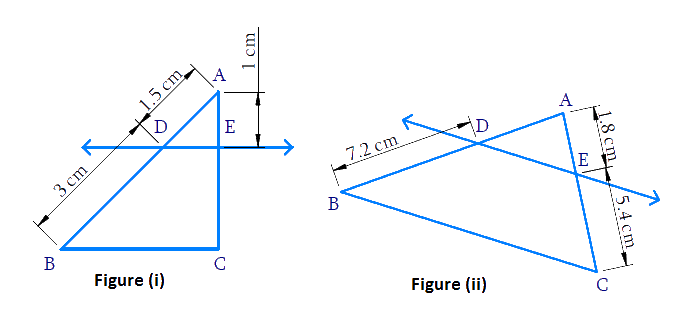### Solution

Reasoning:

As we all know the Basic Proportionality Theorem (B.P.T) or (Thales Theorem)

Two triangles are similar if :

(i) Their corresponding angles are equal

(ii) Their corresponding sides are in the same ratio (or proportion)

Steps:

(i) In$$\,\,\Delta ABC$$

\begin{align} BC||DE \\ \end{align}

In\begin{align}\Delta ABC\,\,&\And \,\,\Delta ADE \end{align}

\begin{align} & \angle ABC=\angle ADE \\ & \left( \because \text{Corresponding}\,\text{angles} \right) \\\\ & \angle ACB=\angle AED \\ & \left( \because \text{Corresponding}\,\text{angles} \right) \\\\ &\quad \;\;\angle A=\angle A \\ &\text{(Common)} \\\\ & \Rightarrow \Delta ABC \sim \Delta \text{ADE} \\ \end{align}

\begin{align} \frac{AD}{DB}&=\frac{AE}{EC} \\ \frac{1.5}{3}&=\frac{1}{EC} \\ EC&=\frac{3\times 1}{1.5} \\ EC&=2\,\text{cm}\end{align}

(ii) Similarly, $$\Delta ABC \sim \Delta ADE$$

\begin{align} \frac{AD}{DB}&=\frac{AE}{EC} \\ \frac{AD}{7.2} &=\frac{1.8}{5.4} \\ AD&=\frac{7.2\times 1.8}{5.4} \\ AD&=2.4\,\text{cm} \\\end{align}

## Chapter 6 Ex.6.2 Question 2

$$E$$ and $$F$$ are points on the sides $$PQ$$ and $$PR$$ respectively of a $$\Delta \mathrm{PQR}$$. For each of the following cases, state whether $$EF || QR:$$

(i)

$$PE = 3.9\,\rm{cm},\; EQ = 3\,\rm{cm},\; PF = 3.6 \,\rm{cm}$$ and $$FR = 2.4\,\rm{cm}$$

(ii)

$$PE = 4\,\rm{cm},\; QE = 4.5\,\rm{cm},\; PF = 8\,\rm{cm}$$ and $$RF = 9\,\rm{cm}$$

(iii)

\begin{align}PQ &= 1.28\,\rm{cm},\;\\ PR &= 2.56 \,\rm{cm}, \\\;PE &= 0.18\,\rm{cm} \\PF &= 0.36\,\rm{cm}\end{align}

### Solution

(i) Reasoning:

As we know that a line divides any two sides of a triangle in the same ratio, then the line is parallel to the third side (converse of $$BPT$$)

Steps: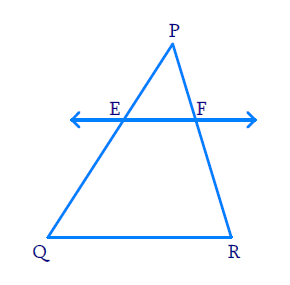Here,

\begin{align} \frac{PE}{EQ}&=\frac{3.9}{3} =1.3\,\text{cm} \\\\ & \rm{and}\\\\ \frac{PF}{FR}&=\frac{3.6}{2.4}= 1.5 \end{align}

Hence,

\begin{align} \frac {{PE}}{EQ} \not= \frac {{PF}}{FR} \end{align}

According to converse of $$BPT,\;EF$$ is not parallel to $$QR$$.

(ii) Reasoning:

As we know that a line divides any two sides of a triangle in the same ratio, then the line is parallel to the third side(converse of $$BPT$$)

Steps:

Here,

\begin{align} \frac{{PE}}{EQ}= \frac{{4}}{4.5}=\frac{{8}}{9} \end{align}

and

\begin{align} \frac{{PF}}{FR}= \frac{{8}}{9} \end{align}

Hence,

\begin{align} \frac{{PE}}{EQ}=\frac{{PF}}{FR} \end{align}

According to converse of $$BPT$$$$EF \parallel QR$$

(iii) Reasoning:

As we know that a line divides any two sides of a triangle in the same ratio, then the line is parallel to the third side(converse of $$BPT$$)

Steps:

Here,

$$PQ=1.28 \,\rm{cm}$$  and $$PE=0.18\,\rm{cm}$$ 

\begin{align} {EQ} &={PQ}-{PE} \\ &=(1.28-0.18)\,\rm{cm} \\ &=1.10\, \mathrm{cm} \end{align}

$$PR=2.56\,\rm{cm}$$ and $$PF=0.36\,\rm{cm}$$

\begin{align} {FR} &={PR}-{PF}\\ &=(2.56-0.36)\,\rm{}cm \\ &=2.20\; \mathrm{cm} \end{align}

Now,

\begin{align} \frac{PE}{EQ}&=\frac{0.18\text{cm}}{1.10\text{cm}} =\frac{18}{110} =\frac{9}{55} \\ \frac{PF}{FR} &=\frac{0.36\text{cm}}{2.20\text{cm}} =\frac{36}{220}=\frac{9}{55} \end{align}

\begin{align}\Rightarrow\frac{PE}{EQ}=\frac{PF}{FR} \end{align}

According to converse of $$BPT,\;EF\parallel QR$$ 

## Chapter 6 Ex.6.2 Question 3

In Figure if $$LM || CB$$ and $$LN || CD$$, prove that

\begin{align}\frac{AM}{AB}=\frac{AN}{AD}\end{align}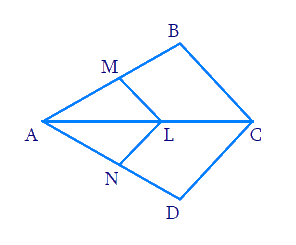### Solution

Reasoning:

As we know if a line is drawn parallel to one side of a triangle to intersect the other two sides in distinct points, the other two sides are divided in the same ratio.

Steps:

In \begin{align} \Delta ABC\end{align}

\begin{align} &LM||CB \\ &\frac{AM}{MB}=\frac{AL}{LC}\;\dots(1) \\ \end{align}

In $$\Delta ACD$$

\begin{align}&LN||CD \\ &\frac{AN}{DN}=\frac{AL}{LC}\;\dots(2) \\ \end{align}

From equations $$(1)$$ and $$(2)$$

\begin{align}\frac{AM}{MB}=\frac{AN}{DN}\end{align}

\begin{align}\Rightarrow \frac{MB}{AM}=\frac{DN}{AN}\end{align}

Adding $$1$$ on both sides

\begin{align} \frac{MB}{AM}+1&=\frac{DN}{AN}+1 \\ \frac{MB+AM}{AM}&=\frac{DN+AN}{AN} \\ \frac{AB}{AM}&=\frac{AD}{AN} \\ \frac{AM}{AB}&=\frac{AN}{AD} \\ \end{align}

## Chapter 6 Ex.6.2 Question 4

In Figure, $$DE || AC$$ and $$DF || AE.$$ Prove that

\begin{align}\frac{BF}{FE}=\frac{BE}{EC}\end{align}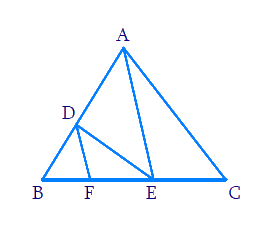### Solution

Reasoning:

As we know if a line is drawn parallel to one side of a triangle to intersect the other two sides in distinct points, the other two sides are divided in the same ratio.

Steps:

In $$\Delta ABC$$

\begin{align} & DE||AC \end{align}

\begin{align}\frac{BD}{AD}=\frac{BE}{EC}\;\dots\text{(i)} \\ \end{align}

In\begin{align}\Delta \,ABE \end{align}

\begin{align}&DF||AE \\ &\frac{BD}{AD}=\frac{BF}{FE}\;\dots\text{(ii)} \end{align}

From $$\rm (i)$$ and $$\rm (ii)$$

\begin{align} & \frac{BD}{AD}=\frac{BE}{EC}=\frac{BF}{FE} \\ & \frac{BE}{EC}=\frac{BF}{FE} \\ \end{align}

## Chapter 6 Ex.6.2 Question 5

In Figure, $$DE || OQ$$ and $$DF || OR.$$ Show that $$EF || QR.$$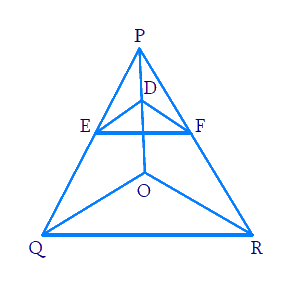### Solution

Reasoning:

As we know if a line divides any two sides of a triangle in the same ratio, then the line is parallel to the third side.

Steps:

In $$\Delta P O Q$$

\begin{align}D E \;&|| O Q \;\text { (Given) } \\ \frac{P E}{E Q}&=\frac{P D}{O D} \;\dots(1)\end{align}

In $$\Delta POR$$

\begin{align}& DF||OR\;\;\left( \text{Given} \right) \\ & \frac{PF}{FR}=\frac{PD}{DO} \;\dots (2) \end{align}

From $$(1)$$$$(2)$$

\begin{align} \frac{PE}{EQ}&=\frac{PF}{FR}=\frac{PD}{DO} \\ \frac{PE}{EQ}&=\frac{PF}{FR} \\ \end{align}

In $$\Delta PQR$$

\begin{align}\frac{PE}{EQ}=\frac{PF}{FR} \end{align}

$$\therefore\;\;QR||EF$$  (Converse of $$BPT$$)

## Chapter 6 Ex.6.2 Question 6

In Figure, $$A, \;B$$ and $$C$$ are points on $$OP, \;OQ$$ and $$OR$$ respectively such that $$AB || PQ$$ and $$AC || PR.$$ Show that $$BC || QR.$$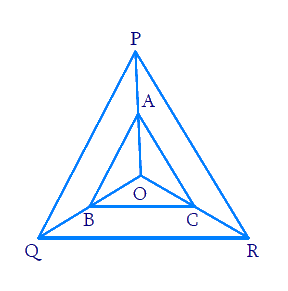### Solution

Reasoning:

As we know if a line divides any two sides of a triangle in the same ratio, then the line is parallel to the third side.

Steps:

In $$\Delta OPQ$$

\begin{align}AB\,&||\,PQ \;\;\text{(Given)}\\ \frac{OA}{AP}&=\frac{OB}{BQ}\;\dots\rm{(i)} \end{align}

$$[\because$$  Thales Theorem (BPT)]

In $$\Delta OPR$$

\begin{align} AC\,&||\,PQ \;\;\text{(Given)} \\ \frac{OA}{AP}&=\frac{OC}{CR}\;\dots\rm{(ii)} \end{align}

[ $$\because$$Thales Theorem ($$BPT$$)]

From $$\rm (i)$$$$\rm (ii)$$

\begin{align}\frac{OA}{AP}=\frac{OB}{BR}=\frac{OC}{CR}\end{align}

\begin{align}\frac{OB}{BQ}=\frac{OC}{CR}\end{align}

Now In $$\Delta OQR$$

\begin{align}\frac{OB}{BQ}=\frac{OC}{CR} \end{align}

$$BC\,||\,QR \;\; [ \because \text{ Converse of BPT} ]$$

## Chapter 6 Ex.6.2 Question 7

Using Theorem $$6.1$$, prove that a line drawn through the midpoint of one side of a triangle parallel to another side bisects the third side. (Recall that you have proved it in Class IX).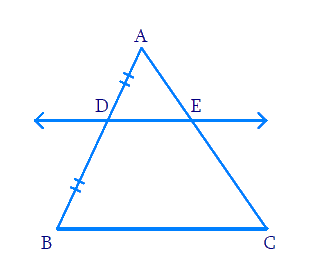### Solution

Reasoning:

We know that theorem $$6.1$$ states that “If a line is drawn parallel to one side of a triangle to intersect the other two sides in distinct points, the other two sides are divided in the same ratio ($$BPT$$)”.

Steps:

In $$\Delta ABC$$$$, D$$ is the midpoint of $$AB$$

Therefore,

$AD = BD$

$\frac{AD}{BD}=1$

Now,

\begin{align}DE&||BC \\ \Rightarrow\frac{AE}{EC}&=\frac{AD}{BD}\,\,\,\text{[Theorem} \,6.1] \\ \Rightarrow \frac{AE}{EC}&=1 \end{align}

Hence $$,E$$ is the midpoint of $$AC.$$

## Chapter 6 Ex.6.2 Question 8

Using Theorem $$6.2$$, prove that the line joining the mid-points of any two sides of a triangle is parallel to the third side. (Recall that you have done it in Class IX).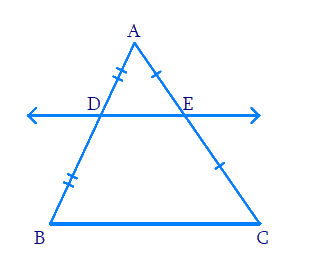### Solution

Reasoning:

We know that theorem $$6.2$$ tells us if a line divides any two sides of a triangle in the same ratio, then the line is parallel to the third side. (Converse of $$BPT$$)

Steps:

In $$\Delta ABC$$

$$D$$ is the midpoint of $$AB$$

\begin{align}AD &= BD\\ \frac{AD}{BD} &= 1\;\dots\rm{(i)}\end{align}

$$E$$ is the midpoint of $$AC$$

\begin{align} AE &= CE\\ \frac{AE}{BE} &= 1\;\dots\rm{(ii)}\end{align}

From (i) and (ii)

\begin{align} \frac{AD}{BD}&=\frac{AE}{BE} = 1 \\ \frac{AD}{BD}&=\frac{AE}{BE} \\\end{align}

According to theorem $$6.2,$$ (Converse of $$BPT$$)

$DE||BC$

## Chapter 6 Ex.6.2 Question 9

$$ABCD$$ is a trapezium in which $$AB || DC$$ and its diagonals intersect each other at the point $$O.$$ Show that \begin{align}\frac{AO}{BO}=\frac{CO}{DO} \end{align}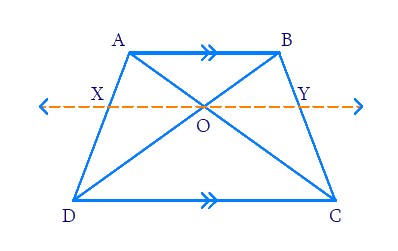### Solution

Reasoning:

As we know if a line divides any two sides of a triangle in the same ratio, then the line is parallel to the third side.

Steps:

In trapezium $$ABCD$$

$$AB||CD,$$  $$AC$$ and $$BD$$ intersect at $$‘O’$$

Construct $$XY$$ parallel to $$AB$$ and $$CD$$ $$(XY\parallel AB,\,(XY \parallel CD)$$ through $$‘O’$$

In \begin{align}\Delta ABC\end{align}

$OY\,||\,AB\;\;(\because \text{Construction})$

According to theorem $$6.1$$ $$(BPT)$$

\begin{align}\frac{BY}{CY}=\frac{OA}{OC}\;\dots\rm{(i)}\end{align}

In $$\Delta BCD$$

$$\rm OY||CD\;\; (\because\, \rm{Construction})$$

According to theorem $$6.1$$ ($$BPT$$)

\begin{align}\frac{BY}{CY}=\frac{OB}{OD}\;\dots\left( \text{ii} \right) \\ \end{align}

From $$\rm (i)$$ and $$\rm (ii)$$

\begin{align} \,\,\,\,\,\,\frac{OA}{OC}&=\frac{OB}{OD}\,\,\,\\ \Rightarrow\quad \frac{OA}{OB}&=\frac{OC}{OD} \\ \end{align}

## Chapter 6 Ex.6.2 Question 10

The diagonals of a quadrilateral $$ABCD$$ intersect each other at the point $$‘O’$$ such that \begin{align}\frac{AO}{BO}=\frac{CO}{DO}\end{align}. Show that $$ABCD$$ is a trapezium.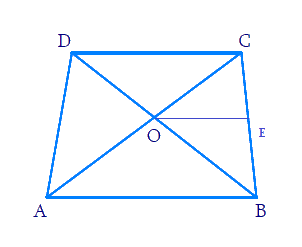### Solution

Reasoning:

As we know if a line divides any two sides of a triangle in the same ratio,then the line is parallel to the third side.

Steps:

In quadrilateral $$ABCD$$

Diagonals $$AC, BD$$ intersect at $$‘O’$$

Draw $$OE||AB$$

In $$\Delta ABC$$

\begin{align}\,\,\,\,\,\,\,\,\,\,\,\,\,\,\,\,\,\,\,\,\,\,\,OE||AB \\ \end{align}

$\Rightarrow \frac{{OA}}{OC} = \frac{{BE}}{CE} \,\,(BPT)\; \cdots(1)$

But \begin{align}\frac{OA}{OB}=\frac{OC}{OD}\,\,\left( \rm{Given} \right)\end{align}

\begin{align}\Rightarrow \frac{{OA}}{OC}=\frac{{OB}}{{OD}}\;\dots(2)\end{align}

From $$(1)$$ and $$(2)$$

\begin{align}\frac{OB}{OD}=\frac{BE}{CE}\,\,\,\end{align}

In $$\Delta BCD$$

\begin{align}\rm \frac{OB}{OD}&=\frac{BE}{CE} \\ \rm OE&\,\,||CD \\ \rm OE& \,\,||AB\,\,\text{and}\,\,\rm OE||CD \\ \Rightarrow \rm AB& \,\,||CD \\ &\!\!\!\!\!\!\!\!\!\!\!\!\!\!\!\!\!\!\Rightarrow \rm ABCD\,\,\text{is a trapezium} \end{align}